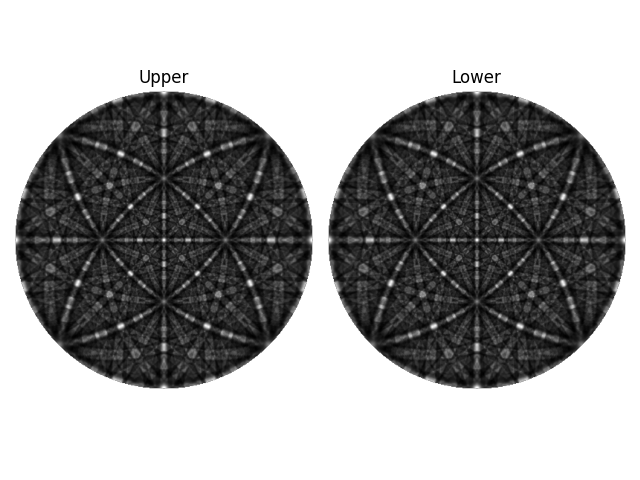# Plot nice master pattern image#

This example shows you how to plot a nice and clean image of an EBSD master pattern.

```import kikuchipy as kp
import matplotlib.pyplot as plt
import numpy as np

mp = kp.data.nickel_ebsd_master_pattern_small(hemisphere="both")
print(mp)
```

Out:

```<EBSDMasterPattern, title: ni_mc_mp_20kv_uint8_gzip_opts9, dimensions: (2|401, 401)>
```

Extract the underlying data of both hemipsheres and mask out the surrounding black pixels

```data = mp.data.astype("float32")
mask = data == 0
data[:, mask] = np.nan
```

Plot both hemispheres with labels

```fig, (ax0, ax1) = plt.subplots(ncols=2)
ax0.imshow(data, cmap="gray")
ax1.imshow(data, cmap="gray")
ax0.axis("off")
ax1.axis("off")
ax0.set_title("Upper")
ax1.set_title("Lower")
fig.tight_layout()
```Total running time of the script: ( 0 minutes 0.862 seconds)

Estimated memory usage: 9 MB

Gallery generated by Sphinx-Gallery